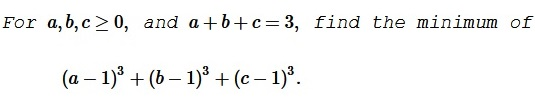# Cubic Optimization with Linear Constraints

### Problem### Solution 1

Let, WLOG, $a\ge b\ge c\ge 0.\,$ Then $\displaystyle a\ge\frac{a+b}{2}\ge\frac{a+b+c}3=1\ge c\,$ such that $a\ge 1,\,$ $a+b\ge 2,\,$ $c\le 1.$

Set $x=a-1,\,$ $y=b-1.\,$ Note that $x+y=a+b-2\ge 0.$

$\displaystyle(a-1)^3+(b-1)^3=x^3+y^3=(x+y)(x^2-xy+y^2).$

We estimate $\displaystyle x^2-xy+y^2\ge\frac{(x+y)^2}{4}\,$ or, equivalently, $4x^2-4xy+4y^2\ge x^2+2xy+y^2,\,$ i.e., $3(x-y)^2\ge 0.\,$ It follows that

\displaystyle\begin{align} x^3+y^3 &= (x+y)(x^2-xy+y^2)\ge\frac{(x+y)^3}{4}\\ &=\frac{(a-1+b-1)^3}{4}=\frac{(1-c)^3}{4}. \end{align}

Consider function $f:\,[0,1]\to\mathbb{R},\,$ defined by $f(c)=\displaystyle -\frac{3}{4}(1-c)^2.\,$ $f'(c)=\displaystyle\frac{9}{4}(1-c)^2\ge 0,\,$ implying that $f\,$ is increasing and, hence $f(c)\ge f(0)=\displaystyle -\frac{3}{4}.\,$ It follows that

$\displaystyle (a-1)^3+(b-1)^3+(c-1)^3\ge -\frac{3}{4}$

which is the minimum, achieved for $c=0\,$ and $\displaystyle a=b=\frac{3}{2}\,$ (and permutations.)

### Solution 2

This is a shortened variant of the above. Assuming $a\ge b\ge c,\,$ $a\ge 1,\,$ $a+b\ge 2,\,$ $c\le 1.$

With $x=a-1,\,$ $y=b-1,\,$ $z=c-1,\,$ $x+y\ge 0\,$ and $x+y+z=0.\,$ we have

\displaystyle\begin{align} &(a-1)^3+(b-1)^3+(c-1)^3 = x^3+y^3+z^3\\ &\qquad\qquad\qquad=3xyz+(x+y+z)(x^2+y^2+z^2-xy-yz-zx)\\ &\qquad\qquad\qquad=3xyz=-3xy(x+y). \end{align}

Now, since $\displaystyle xy\le\frac{(x+y)^2}{4}\,$ and $x+y\ge 0,\,$ we get

$\displaystyle -3xy(x+y)\ge -\frac{3}{4}(x+y)^3=-\frac{3}{4}(1-c)^3\ge -\frac{3}{4},$

because $1\ge c\ge 0.\,$ The minimum is attained for $c=0\,$ and $\displaystyle a=b=\frac{3}{2}\,$ (and permutations.)

### Solution 3

With the reference to Vo Quoc Ba Can's theory, we introduce $p=a+b+c,\,$ $ab+bc+ca=\displaystyle\frac{p^2-q^2}{3},\,$ $q\ge 0,\,$ and $r=abc.\,$ Let $E=\displaystyle\sum_{cycl}(a-1)^3.\,$ The constraint informs us that $\displaystyle\sum_{cycl}(a-1)=0\,$ and, subsequently, $\displaystyle E=3\prod_{cycl}(a-1),$ such that $\displaystyle E=3\left(r-\sum_{cycl}ab+2\right).$

We know that

$\displaystyle r\ge\frac{1}{27}(p+q)^2(p-2q)=\frac{1}{27}(3+q)^2(3-2q)\ge 0,$

implying $\displaystyle q\le\frac{3}{2}\,$ and

\displaystyle\begin{align}\frac{E}{3}&=r-\sum_{cycl}ab+2\\ &\ge\frac{1}{27}(3+q)^2(3-2q)-\frac{9-q^2}{3}+2\\ &=-\frac{2q^3}{27}\ge-\frac{2}{27}\left(\frac{3}{2}\right)^3=-\frac{1}{4}, \end{align}

i.e., $\displaystyle E\ge-\frac{3}{4}.\,$ Equality (an, hence $\min E\,)$ is attained at $\displaystyle q=\frac{3}{2},\,$ with $\displaystyle\sum_{cycl}ab=\frac{9}{4}\,$ and $r=0,\,$ implying $\displaystyle (a,b,c)=\left(\frac{3}{2},\frac{3}{2},0\right)\,$ and the permutations.

### Solution 4

We'll look for the minimum of $x^3+y^3+z^3,\,$ provided $x,y,z\ge -1\,$ and $x+y+z=0.$

WLOG, $x\le y\le z.\,$ Observe that the function $f(t)=t^3\,$ is concave on $[-1,0]\,$ and convex on $[0,\infty).\,$ We'll consider two cases.

Case 1: $-1\le x\le y\le 0$

According to Karamata's theorem, $f(x+y)+f(0)\le f(x)+f(y),\,$ implying $(x+y)^3\le x^3+y^3,\,$ i.e., $(x+y)^3+z^3\le x^3+y^3+z^3.\,$ We conclude that $x^3+y^3+z^3\ge 0.$

Case 2: $0\le y\le z$

By Jensen's inequality, $\displaystyle f(y)+f(z)\ge 2f\left(\frac{y+z}{2}\right),\,$ such that $\displaystyle y^3+z^3\ge 2\left(-\frac{x}{2}\right)^3,\,$ i.e., $x^3+y^3+z^3\ge\displaystyle\frac{3x^3}{4}.\,$ But $\displaystyle\frac{3x^3}{4}\ge -\frac{3}{4}.\,$ Note that is $x=-1\,$ and $\displaystyle y=z=\frac{1}{2},\,$ equality is attained: $\displaystyle x^3+y^3+z^3=-\frac{3}{4}.\,$ Thus, we deduce that $\displaystyle\min (x^3+y^3+z^3)=-\frac{3}{4}.$

### Solution 5

Note that $\displaystyle\sum_{cycl}(a-1)^3=3abc-3(ab+bc+ca)+6\,$ and consider two cases:

Case 1: $4(ab+bc+ca)\le 9$

In this case, we have

$\displaystyle\sum_{cycl}(a-1)^3\ge 0-\frac{27}{4}+6=-\frac{3}{4}.$

Case 2: $4(ab+bc+ca)\ge 9$

In this case, using Schur's inequality,

\displaystyle\begin{align} \sum_{cycl}(a-1)^3&\ge [4(ab+bc+ca)-9]-3(ab+bc+ca)+6\\ &\ge (ab+bc+ca)-3\\ &ge -\frac{3}{4}. \end{align}

Equality holds when $\displaystyle a=b=\frac{3}{2}\,$ and $c=0,\,$ or any cyclic permutations.

### Acknowledgment

Marian Dincă has kindly messaged me his two solutions to a problem posted to the Imad Zak facebook group by AD Nguyen. Solution 3 is by Imad Zak; Solution 4 is by Leo Giugiuc; Solution 5 is by Le Khanhsy.

### A Sample of Optimization Problems

• Mathematicians Like to Optimize
• Reshuffling knights - castle defenders
• Isoperimetric Theorem and Inequality
• Viewing a Statue: the Problem of Regiomontanus
• Fagnano's Problem
• Minimax Principle Demonstration
• Maximum Perimeter Property of the Incircle
• Extremal Problem in a Circular Segment
• Optimization in Four Variables with Two Constraints
• Daniel Dan's Optimization in Three Variables
• Problem in a Special Trapezoid
• Cubic Optimization with Partly Linear Constraints
• Problem M317 from Crux Mathematicorum
• Find the Maximum and Minimum of a Function
• Area of Isosceles Triangle
• Minimum of Cotangents from Saint Petersburg
•